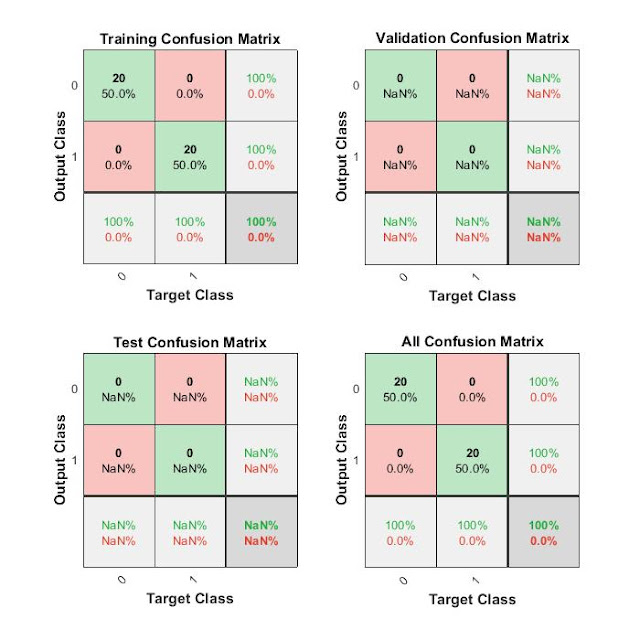## Wednesday, 31 October 2018

### Calculate neuron output,Plot perceptron input/target vectors

Train net and calculate neuron output
Plot perceptron input/target vectors
>> N = 20;
% define inputs and outputs
offset = 5; % offset for second class
x = [randn(2,N) randn(2,N)+offset]; % inputs
y = [zeros(1,N) ones(1,N)]; % outputs
% Plot input samples with PLOTPV (Plot perceptron input/target vectors)
figure(1)
plotpv(x,y);
>> net = perceptron;
net = train(net,x,y);
view(net);
>> figure(1)
plotpc(net.IW{1},net.b{1});Add caption

# How to record sound and do spectral analysis in Matlab ,

>> audiorecorder(8000,8,1);
>> a=audiorecorder(8000,8,1);
>> record(a,10);
>> b=getaudiodata(a);
>> play(b,8000);
Undefined function 'play' for input arguments of type 'double'.

>> sound(b);
>> plot(b);
>> e=fft(b);
>> plot(e);
>> plot(abs(e));
>> plot(abs(e));

## Previous year question paper,quantitative analysis

### Latin Cubes

What is Latin Cubes? The practical applications of Latin crops and related designs are factorial experiment.factorial experiments for me...